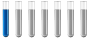## Reaction heat of a LiNiMnCoO2 (NMC) Lithium-Ion Battery

Chemistry and homework help forum.

Organic Chemistry, Analytical Chemistry, Biochemistry, Physical Chemistry, Computational Chemistry, Theoretical Chemistry, High School Chemistry, Colledge Chemistry and University Chemistry Forum.

Share your chemistry ideas, discuss chemical problems, ask for help with scientific chemistry questions, inspire others by your chemistry vision!

Please feel free to start a scientific chemistry discussion here!

Discuss chemistry homework problems with experts!

Ask for help with chemical questions and help others with your chemistry knowledge!

Moderators: expert, ChenBeier, Xen

Maxx92
NewbiePosts: 1
Joined: Wed Sep 27, 2017 8:37 am

### Reaction heat of a LiNiMnCoO2 (NMC) Lithium-Ion Battery

Hello,

I'm trying to calculate the reversible reaction heat during charging and discharging of a Lithium-Ion Battery. Additionally Im trying to calculate the irreversible reaction heat of a battery that occurs as a result of high current.

The positive active material of the Battery is LiNiMnCoO2 (NMC).
The reaction equalation ist (M = Metal: Ni,Mn,Co):For the reversible heat during charging and discharging applies Q = dS * T. To calculate dS I need the Standard reaction enthalpy and the Gibbs free energy for Li Ni Mn Co O2. I would do it like in this pictue:Here is an other example for calculating the heat during charging and discharging of a Nickel-Metalhybrid (NiMH) battery:Result:

dS * T for 25 Â°C Q1 = dS * T = 29,3 kJ/H2 mol

They also calculated the irreversible heat, as a result of reaction of the electrolyt with the electrons occurs as a result of high current:Results:
Standard reaction enthalpy dH = -285,9 kJ/mol
And for the reversible heat T * dS = -48,6 kJ/mol (bei 25 Â°C)

They also use this equalation, I'm not sure why:To calculate the reaction heat that occurs as result of high current, they used dH:

Qreact = dH

Gibbs free energy: dG = dH - dS * T.

dS * T describes the Transformation of during charging and discharging emerging Energie in heat and dH the Transformation of the energy in mechanical work. I think this is the reason why they used dH.

Chemistry is not my strongest skill, so i would be glad, if someone could help me.

Kind regards Maxx